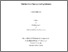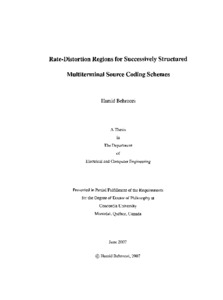Title:

# Rate-distortion regions for successively structured multiterminal source coding schemes

Behroozi, Hamid (2007) Rate-distortion regions for successively structured multiterminal source coding schemes. PhD thesis, Concordia University.Preview
Text (application/pdf)
NR31141.pdf - Accepted Version
4MB

## Abstract

Multiterminal source coding refers to separate encoding and joint decoding of multiple correlated sources. Joint decoding requires all the messages to be decoded simultaneously which is exponentially more complex than a sequence of single-message decodings. Inspired by previous work on successive coding strategy, which is based on successive decoding structure, we apply the successive Wyner-Ziv coding to different schemes of multiterminal source coding problem. We address the problem from an information theoretic perspective and determine the rate region for three different multiterminal coding schemes: Gaussian CEO problem, 1-helper problem, and 2-terminal source coding problem. We prove that the optimal sum-rate distortion performance for the CEO problem is achievable using the successive coding strategy which is essentially a low complexity approach for obtaining a prescribed distortion. We show that if the sum-rate tends to infinity for a finite number of agents (sensors), the optimal rate allocation strategy assigns equal rates to all agents. The same result is obtained when the number of agents tends to infinity while the sum-rate is finite. Then, we consider 1-helper source coding scheme where one source provides partial side information to the decoder to help the reconstruction of the other source. Our results show that the successive coding strategy is an optimal strategy in this scheme in the sense of achieving the rate-distortion function. For the 2-terminal source coding problem, we develop connections between source encoding and data fusion steps and prove that the whole rate-distortion region is achievable using the successive coding strategy. Comparing the performance of the sequential coding with the performance of the successive coding, we show that there is no sum-rate loss when the side information is not available at the encoder. This result is of special interest in some applications such as video coding where there are processing and storage constraints at the encoder. Based on the successive coding strategy, we provide an achievable rate-distortion region for the m-terminal source coding. We also consider a distributed network, modeled by CEO problem with Gaussian multiple access channel (MAC), where L noisy observations of a memoryless Gaussian source are transmitted through an additive white Gaussian MAC to a decoder. The decoder wishes to reconstruct the main source with an average distortion D at the smallest possible power consumption in the communication link. Our goal is to characterize the power-distortion region achievable by any coding strategy regardless of delay and complexity. We obtain a necessary condition for achievability of all power-distortion tuples ( P 1 , P 2 ,..., P L , D ). Also, analyzing the uncoded transmission scheme provides a sufficient condition for achievability of ( P 1 , P 2 ,..., P L , D ). Then, we consider a symmetric case of the problem where the observations of agents have the same noise level and the transmitting signals are subject to the same average power constraint. We show that in this case the necessary and sufficient conditions coincide and give the optimal power-distortion region. Therefore, in the symmetric case of Gaussian CEO problem uncoded transmission over a Gaussian MAC performs optimally for any finite number of agents

Divisions: Concordia University > Gina Cody School of Engineering and Computer Science > Electrical and Computer Engineering Thesis (PhD) Behroozi, Hamid xvi, 110 leaves : ill. ; 29 cm. Concordia University Ph. D. Electrical and Computer Engineering 2007 Soleymani, M. Reza LE 3 C66E44P 2007 B44 975372 Concordia University Library 22 Jan 2013 16:06 13 Jul 2020 20:07 https://concordiauniversity.on.worldcat....
All items in Spectrum are protected by copyright, with all rights reserved. The use of items is governed by Spectrum's terms of access.

Repository Staff Only: item control pageResearch related to the current document (at the CORE website)
Back to top